Courses

# NCERT Solutions(Part- 3)- Data Handling Class 8 Notes | EduRev

## Mathematics (Maths) Class 8

Created by: Full Circle

## Class 8 : NCERT Solutions(Part- 3)- Data Handling Class 8 Notes | EduRev

The document NCERT Solutions(Part- 3)- Data Handling Class 8 Notes | EduRev is a part of the Class 8 Course Mathematics (Maths) Class 8.
All you need of Class 8 at this link: Class 8

Question 1. If you try to start a scooter, what are the possible outcomes?

Solution: It may start. It may not start.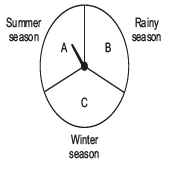Question 2. When a die is thrown what are the possible outcomes?

Solution: The possible out comes are: 1, 2, 3, 4, 5 or 6.

Question 3. When you spin the wheel shown, what are the possible outcomes?

Solution: The possible outcomes are A, B or C.

Question 4. You have a bag with five identical balls of different colours and you are to pull out (draw) a ball without looking at it; list the outcomes you would get.

Solution: The possible outcomes are: W, R, B, G or Y.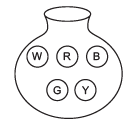Question 5: In throwing a die:

(a) Does the first player have a greater chance of getting a six?

(b) Would the player who played after him have a lesser chance of getting a six?

(c) Suppose the second player got a six. Does it mean that the third player would not have a chance of getting a six?

Solution: (a) No (b) No (c) No

Question 6: Suppose you spin the wheel.

1.

(i) List the number of outcomes of getting a green sector and not getting a green sector on this wheel.

(ii) Find the probability of getting a green sector.

(iii) Find the probability of not getting a green sector.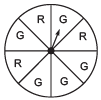Solution:

(i) Number of outcomes of getting a green sector = 5

Number of outcomes of not getting a green sector = 3

(ii) ∵ The total number of equally likely outcomes = 8

Number of outcomes of getting a green sector = 5

∴ Probability of getting a green sector = 5/8

(iii) Probability of getting a not-green sector = 3/8

Exercise 5.3

Question 1. List the outcomes you can see in these experiments.

(a) Spinning a wheel

(b) Tossing two coins together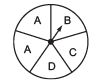Solution:

(a) Outcomes is spinning the given wheel are: A, B, C or D.

(b) Outcomes in tossing two coins together are: HT, HH, TH, TT

[where HT → Head on first coin + Tail on second coin and so on]

Question 2. When a die is thrown, list the outcome of an event of getting

(i) (a) a prime number   (b) not a prime number

(ii) (a) a number greater than 5   (b) a number not greater than 5.

Solution: Possible outcomes are: 1, 2, 3, 4, 5 or 6.

Out of them prime numbers are 2, 3 and 5.

(i) (a) Outcomes of getting a prime number are: 2, 3 or 5.

(b) Outcomes of getting not a prime number are: 1, 4 or 6

(ii) (a) Outcome of getting a number greater than 5 is 6.

(b) Outcomes of getting a number not greater than 5 are: 1, 2, 3, 4 or 5.

Question 3. Find:

(a) the probability of the pointer stopping on D in (Question 1-(a))?

(b) the probability of getting ace from a well shuffled deck of 52 playing cards?

(c) the probability of getting a red apple. (See figure below)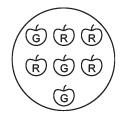Solution: (a) On the spinning wheel there are 5 sectors containing A, B, C and D.

Since, there is only 1 sector containing D.

i.e. possible outcome = 1

Number of equally likely outcomes = 5

∴Probability = 1/5

(b) Number of possible outcomes = 52

Since, there are 4 aces in a pack of 52 cards, and out of them one ace can be obtained in 4 ways.

∴ Equally likely outcomes = 4

∴ Probability of getting an ace = 4/52 = 1/13

(c) There are 7 apples in all

∴ Possible number of ways = 7

Since, there are 4 red apples: Therefore, a red apple can be obtained in 4 ways.

i.e. Equally likely outcome = 4

∴ Probability of getting a red apple = 4/7

Question 4. Numbers 1 to 10 are written on ten separate slips (one number on one slip), kept in a box and mixed well. One slip is chosen from the box without looking into it. What is the probability of:

(i) getting a number 6?

(ii) Getting a number less than 6?

(iii) Getting a number greater than 6?

(iv) Getting a 1-digit number?

Solution: Since, there are 10 slips. At a time any slip can be taken out.

∴ Number of total outcomes = 10

Now,

(i) We can get a slip containing the number ‘6’ only once.

∴ Number of favourable outcome = 1

Thus, probability of getting the number 6 = 1/10

(ii) There are five numbers (i.e. 1, 2, 3, 4 and 5) which are less than 6.

∴Favourable outcomes are 5.

Thus, Probability of getting a number less than six = 5/10 = 1/2

(iii) There are 4 numbers (i.e. 7, 8, 9, 10) which are greater than six.

∴ Favorable outcomes = 4

Thus, probability of getting a number greater than six =  4/10 = 2/5

(iv) There nine one-digit numbers i.e. (1, 2, 3, 4, 5, 6, 7, 8 and 9)

∴ Favorable outcomes = 9

Thus, probability of getting a one-digit number = 9/10

Question 5. If you have a spinning wheel with 3 green sectors, 1 blue sector and 1 red sector, what is the probability of getting a green sector? What is the probability of getting a non-blue sector?

Solution: There are 5 sectors in all (3 green + 1 blue + 1 red).

∴There are total possible outcomes = 5.

Since, there are 3 green sectors.

i.e. Number of favourable outcomes = 3

Thus, probability of getting a green sector =  3/5

Again, there are 4 non-blue sectors

∴ Favouable outcomes = 4

Thus, probability of getting a ‘non-blue’ sector =  4/5

Question 6. Find the probabilities of the events given in Question 2.

Solution: There are 6 outcomes in all (i.e. 1, 2, 3, 4, 5, 6)

(i) Since, there are 3 prime numbers (i.e. 2, 3, and 5)

∴ Favourable outcomes = 3

Thus, probability of getting a prime number =  3/6 = 1/2

(ii) Since, there are 3 non-prime numbers (i.e. 1, 4 and 6)

∴ Favourable outcomes = 3

Thus, the probability of getting a non-prime number = 3/6 = 1/2

(iii) Since, there is 1 number greater than 5 (i.e. 6)

∴ Favourable outcome = 1

Thus, probability of a number greater than 5 = 1/6

(iv) Since, there are 5 numbers which are not greater than 5 (i.e. 1, 2, 3, 4 and 5)

∴ Number of favourable outcomes = 5

Thus, the probability of a number which is not greater than 5 = 5/6

,

,

,

,

,

,

,

,

,

,

,

,

,

,

,

,

,

,

,

,

,

;